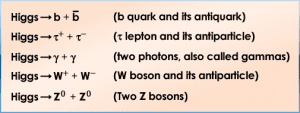Topics: AI | Data Science | Data Visualization | Deep Learning | Machine Learning | NLP | Python | R | Statistics

KDnuggets Home » News » 2018 » Jan » Tutorials, Overviews » Quantum Machine Learning: An Overview ( 18:n02 )

#Quantum Machine Learning: An Overview

Quantum Machine Learning (Quantum ML) is the interdisciplinary area combining Quantum Physics and Machine Learning(ML). It is a symbiotic association- leveraging the power of Quantum Computing to produce quantum versions of ML algorithms, and applying classical ML algorithms to analyze quantum systems. Read this article for an introduction to Quantum ML.

• Quantum methods to improve the Higgs Boson experiment:

In the Higgs Boson experiment, the Higgs boson particle decays to various collections of less massive particles almost instantly after they are produced. The most common decays (among those we are capable of seeing) are those to:(Source)

-signal: 4-lepton events that will occur from decays of a Higgs boson.

-background : 4-lepton events that will occur from decays not involving a Higgs boson.

Attempts were made to separate the signals from the background in solving the 'Higgs-signal-versus-background' ML optimization problem. This study found that using a mixture of quantum annealing and classical annealing was advantageous over state-of-the-art ML methods.

•  Quantum algorithm to solve linear systems:

Some quantum techniques are also helpful in solving subroutines in ML problems, such as matrix inversion.

With the problem stated as given a matrix A and a vector b, find a vector x such that Ax=b. For the quantum algorithm to solve a linear system of equations, we do not need to know the solution x itself, but rather an approximation of the expectation value of some operator associated with x, e.g., x'Mx for some matrix M.

2b) Classical ML to analyze quantum systems

Classical ML algorithms have been used to harness, control and benchmark systems exhibiting quantum phenomena such as Bose-Einstein condensates (BEC’s).

BEC’s are a state of matter where a dilute gas of bosons is cooled to temperatures very close to absolute zero. In BEC’s, most bosons occupy the ground state. Quantum effects usually disappear on a macroscopic level, but BEC’s show visible quantum effects on a macroscopic level. Scientists have created a machine learner that discovers an optimal evaporation ramp for the creation of high-quality BEC’s. Additionally, the process of optimized BEC creation can be understood better because the learned machine learning model determines which parameters are essential in the creation of BEC’s.

There are several building blocks in the design of quantum devices that ML algorithms have aided in. ML algorithms have helped in problems such as:

A quantum device can emit particles in a certain state, and at some unknown point, begin to emit particles in a different state . To detect this change point, scientists compared the performance of local measurements* with that of a global measurement**.

*local measurement: measuring the state of each particle as soon as it arrives at the detector.

**global measurement: waiting until all of the particles have reached the detector and making a measurement at the very end

For local measurements, Sasaki framed the classification of unknown states as a form of supervised learning. In the end, it was found that global measurements outperform local measurements in detecting sudden quantum changes.

Scientists trained a quantum learning machine to perform binary classification of qubit states into 0 and 1, with the classical memory growing only logarithmically with the number of training qubits. It performs well even under a changing composition of a large-enough training set.

• Quantum decoherence:

When the quantum system is not perfectly isolated, there is a loss of information from the system into its environment. This is called quantum decoherence and leads to the loss of quantum behavior. ML techniques were employed to learn more about the stochastic dephasing process on a qubit, to predict qubit decoherence and stabilize against future dephasing of qubits.

Thermodynamic observables such as energy, specific heat and magnetism were faithfully reproduced using a recurrent neural network called a Boltzmann machine. The machine was trained using datasets generated by Monte Carlo sampling.

Related:

Top Stories Past 30 Days
Most Popular
Most SharedGet KDnuggets, a leading newsletter on AI, Data Science, and Machine Learning